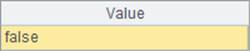# 条形码检查和校验 (#60)

l  问题

l  思路

l  代码

 A 1 ="1234567890123" 给出的条形码 2 =A1. split@p() 将条形码转化为序列 3 =A2(to(12)).step(2,1).sum() 前12位奇数位的数字之和 4 =A2(to(12)).step(2,2).sum() 计前12位偶数位的数字之和 5 =abs((A3-A4)%10) 奇数位之和减去偶数位之和再对10求余最后取绝对值 6 =A5==A2.m(-1) 判断A5的结果是否与条形码的最后一位相同

l  结果